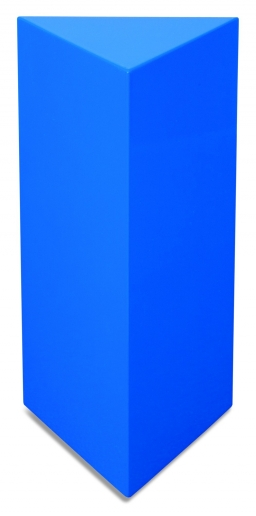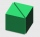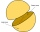# Base of prism

The base of the perpendicular prism is a rectangular triangle whose legs length are at a 3: 4 ratio. The height of the prism is 2cm smaller than the larger base leg. Determine the volume of the prism if its surface is 468 cm2.

Correct result:

V =  540 cm3

#### Solution:

$S=468 \ \text{cm}^2 \ \\ a:b=3:4 \ \\ h=b-2 \ \\ \ \\ a^2 + b^2=c^2 \ \\ a=3x \ \\ b=4x \ \\ c=5x \ \\ 3^2+4^2=5^2 \ \\ \ \\ \ \\ S=ab + (a+b+c)h \ \\ S=3 \cdot \ 4 \cdot \ x^2 + (3x+4x+5x)(4x-2) \ \\ \ \\ 3*4*x^2 + (3x+4x+5x)(4x-2)=468 \ \\ \ \\ 3 \cdot \ 4 \cdot \ x^2 + (3x+4x+5x)(4x-2)=468 \ \\ 60x^2 -24x -468=0 \ \\ \ \\ a=60; b=-24; c=-468 \ \\ D=b^2 - 4ac=24^2 - 4\cdot 60 \cdot (-468)=112896 \ \\ D>0 \ \\ \ \\ x_{1,2}=\dfrac{ -b \pm \sqrt{ D } }{ 2a }=\dfrac{ 24 \pm \sqrt{ 112896 } }{ 120 } \ \\ x_{1,2}=\dfrac{ 24 \pm 336 }{ 120 } \ \\ x_{1,2}=0.2 \pm 2.8 \ \\ x_{1}=3 \ \\ x_{2}=-2.6 \ \\ \ \\ \text{ Factored form of the equation: } \ \\ 60 (x -3) (x +2.6)=0 \ \\ \ \\ x>0 \ \\ x=x_{1}=3 \ \text{cm} \ \\ \ \\ a=3 \cdot \ x=3 \cdot \ 3=9 \ \text{cm} \ \\ b=4 \cdot \ x=4 \cdot \ 3=12 \ \text{cm} \ \\ c=5 \cdot \ x=5 \cdot \ 3=15 \ \text{cm} \ \\ h=b-2=12-2=10 \ \text{cm} \ \\ \ \\ S_{2}=a \cdot \ b + (a+b+c) \cdot \ h=9 \cdot \ 12 + (9+12+15) \cdot \ 10=468 \ \text{cm}^2 \ \\ S_{2}=S \ \\ \ \\ V=\dfrac{ a \cdot \ b }{ 2 } \cdot \ h=\dfrac{ 9 \cdot \ 12 }{ 2 } \cdot \ 10=540 \ \text{cm}^3$

Checkout calculation with our calculator of quadratic equations.Our examples were largely sent or created by pupils and students themselves. Therefore, we would be pleased if you could send us any errors you found, spelling mistakes, or rephasing the example. Thank you!

Leave us a comment of this math problem and its solution (i.e. if it is still somewhat unclear...):Be the first to comment!Tips to related online calculators
Pythagorean theorem is the base for the right triangle calculator.
Tip: Our volume units converter will help you with the conversion of volume units.

#### You need to know the following knowledge to solve this word math problem:

We encourage you to watch this tutorial video on this math problem:

## Next similar math problems:

• Triangular prismThe base of the perpendicular triangular prism is a rectangular triangle with a hypotenuse of 10 cm and one leg of 8 cm. The prism height is 75% of the perimeter of the base. Calculate the volume and surface of the prism.
• Triangular prism - regularThe regular triangular prism is 7 cm high. Its base is an equilateral triangle whose height is 3 cm. Calculate the surface and volume of this prism.
• Triangular prismCalculate the surface of a triangular prism with the base of an equilateral triangle with a side length of 7.5 cm and a corresponding height of 6.5 cm. Prism height is 15cm.
• Triangular prismCalculate the surface of a regular triangular prism, the edges of the base are 6 cm long and the height of the prism is 15 cm.The surface of the regular quadrilateral prism is 8800 cm2, the base edge is 20 cm long. Calculate the volume of the prism
• Pentagonal prismThe regular pentagonal prism is 10 cm high. The radius of the circle of the described base is 8 cm. Calculate the volume and surface area of the prism.The quadrilateral pyramid has a rectangular base of 24 cm x 3.2dm and a body height of 0.4m. Calculate its volume and surface area.Calculate the surface of a quadrilateral pyramid, which has a rectangular base with dimensions a = 8 cm, b = 6 cm and height H = 10 cm.
• The tentCalculate how much cover (without a floor) is used to make a tent that has the shape of a regular square pyramid. The edge of the base is 3 m long and the height of the tent is 2 m.
• Hexagonal pyramidCalculate the surface area of a regular hexagonal pyramid with a base inscribed in a circle with a radius of 8 cm and a height of 20 cm.In a regular quadrilateral pyramid, the side edge is e = 7 dm and the diagonal of the base is 50 cm. Calculate the pyramid shell area.Determine the surface of a regular tetrahedral pyramid when its volume is V = 120 and the angle of the sidewall with the base plane is α = 42° 30´.The regular triangular prism, whose edges are identical, has a surface of 2514 cm ^ 2 (square). Find the volume of this body in cm3 (l).In a wooden hemisphere with a radius r = 1, a hemispherical depression with a radius r/2 was created so that the bases of both hemispheres lie in the same plane. What is the surface of the created body (including the surface of the depression)?The rectangle with dimensions 8 cm and 4 cm is rotated 360º first around the longer side to form the first body. Then, we similarly rotate the rectangle around the shorter side b to form a second body. Determine the ratio of surfaces of the first and secoThe hollow cylinder has a height of 70 cm, an outer diameter of 180 cm and an inner diameter of 120 cm. What is the surface of the body, including the area inside the cavity?3750 cm square of wallpaper is needed to glue a cube-shaped box. Can Dad cut out the whole necessary piece of wallpaper as a whole if he has a roll of wallpaper 50 cm wide?